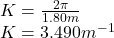## A transverse sine wave with an amplitude of 2.50 mm and a wavelength of 1.80 m travels from left to right along a long, horizontal stretched

Question

A transverse sine wave with an amplitude of 2.50 mm and a wavelength of 1.80 m travels from left to right along a long, horizontal stretched string with a speed of 36.0 m/s. Take the origin at the left end of the undisturbed string. At time t = 0, the left end of the string has its maximum upward displacement.

(a) What are the frequency and angular frequency of the wave?

(b) What is the wave number of the wave?

in progress 0
2 months 2021-09-02T05:23:05+00:00 1 Answers 13 views 0

(a)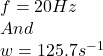(b)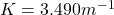Explanation:

We know that the speed of any periodic wave is given by:

v=f×λ

The wave number k is given by:

K=2π/λ

Given data

Amplitude A=2.50mm

Wavelength λ=1.80m

Speed v=36 m/s

For Part (a)

For the wave frequency we plug our values for v and λ.So we get:

v=f×λ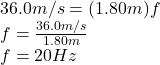And the angular speed we plug our value for f so we get: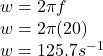For Part (b)

For wave number we plug the value for λ.So we get

K=2π/λ